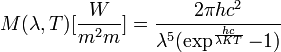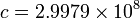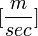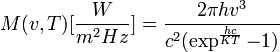A black body absorbs and then re-emits all incident EM radiation. By definition it has an absorptivity and emissivity of 1, and a transmissivity and reflectivity of 0. The Planck Black Body equation describes the spectral exitance of an ideal black body. The study of black-body radiation was an integral step in the formulation of quantum mechanics.

## Contents

### Planck's Law: Wavelength

Formulated in terms of wavelength:$M(\lambda,T) [\frac{W}{m^2 m}] = \frac{ 2 \pi h c^2 }{ \lambda^5 ( \exp^{\frac{h c}{\lambda K T}} - 1 ) }$

where:

Symbol Units Description
λ [m] Input wavelength
T [K] Input temperature$h = 6.6261 \times 10^{-34}$ [J * s] Planck's constant$c = 2.9979 \times 10^{8}$$[\frac{m}{sec}]$ Speed of light in vacuum$k = 1.3807 \times 10^{-23}$ [erg * K] Boltzmann constant

Note that the input λ is in meters and that the output is a spectral irradiance in [W / m2 * m]. Omitting the π term from the numerator gives the blackbody emission in terms of radiance, with units [W / m2 * sr * m] where "sr" is steradians.

### Planck's Law: Frequency

Formulated in terms of frequency:$M(v,T) [\frac{W}{m^2 Hz}] = \frac{ 2 \pi h v^3 }{ c^2 ( \exp^{\frac{h c}{K T}} - 1 ) }$

where:

Symbol Units Description
v [Hz] Input frequency

All other units are the same as for the Wavelength formulation. Again, dropping the π from the numerator gives the result in radiance rather than irradiance.

### Properties of the Planck Equation

Taking the first derivative leads to the wavelength with maximum exitance. This is known as the Wien Displacement Law.

A closed form solution exists for the integral of the Planck blackbody equation over the entire spectrum. This is the Stefan-Boltzmann equation. In general, there is no closed-form solution for the definite integral of the Planck blackbody equation; numerical integration techniques must be used.

The relationship between the ideal blackbody exitance and the actual exitance of a surface is given by emissivity.

An ideal blackbody at 300K (~30 Celsius) has a peak emission 9.66 microns. It has virtually no self-emission before 2.5 microns, hence self-emission is typically associated with the "thermal" regions of the EM spectrum. However, the Sun can be characterized as a 5900K blackbody and has a peak emission around 0.49 microns which is in the visible region of spectrum.

The Planck equation has a single maximum. The wavelength with peak exitance becomes shorter as temperature increases. The total exitance increases with temperature.